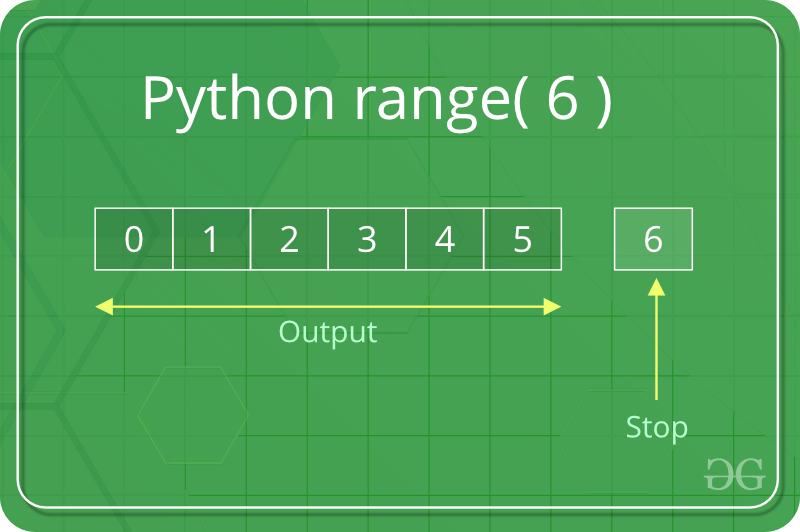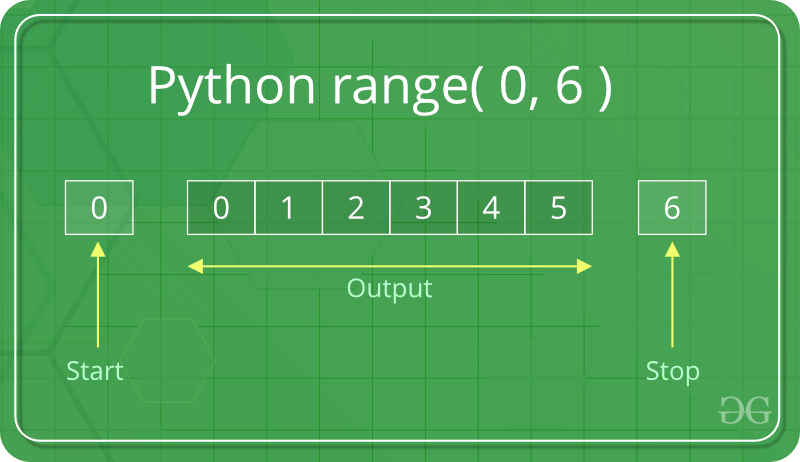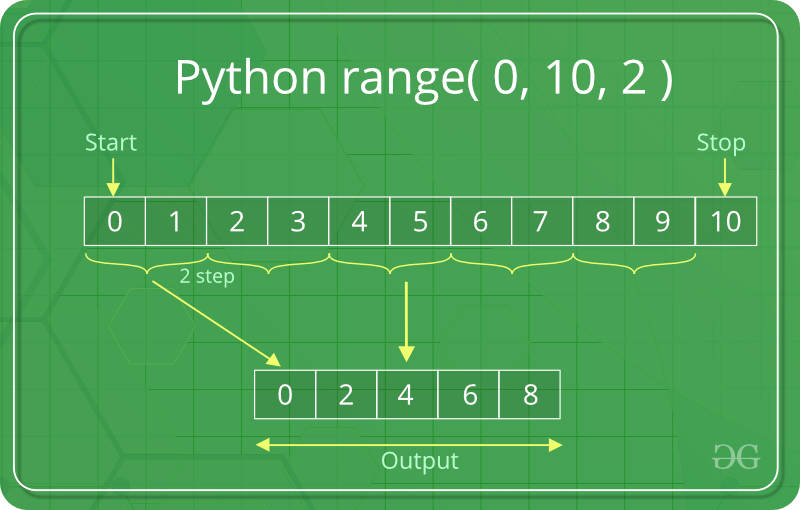GeeksforGeeks App
Open AppBrowser
Continue

# Python range() function

The Python range() function returns a sequence of numbers, in a given range. The most common use of it is to iterate sequence on a sequence of numbers using Python loops.

## Syntax of Python range() function

Syntax: range(start, stop, step)

Parameter:

• start: [ optional ] start value of the sequence
• stop: next value after the end value of the sequence
• step: [ optional ] integer value, denoting the difference between any two numbers in the sequence.

Return: Returns a range type object.

## Python3

 `# print first 5 integers``# using python range() function``for` `i ``in` `range``(``5``):``    ``print``(i, end``=``" "``)``print``()`

Output:

`0 1 2 3 4 `

## What is the use of the range function in Python

In simple terms, range() allows the user to generate a series of numbers within a given range. Depending on how many arguments the user is passing to the function, the user can decide where that series of numbers will begin and end, as well as how big the difference will be between one number and the next. Python range() function takes can be initialized in 3 ways.

• range (stop) takes one argument.
• range (start, stop) takes two arguments.
• range (start, stop, step) takes three arguments.

## Python range (stop)

When the user call range() with one argument, the user will get a series of numbers that starts at 0 and includes every whole number up to, but not including, the number that the user has provided as the stop.Python range visualization

## Python3

 `# printing first 6``# whole number``for` `i ``in` `range``(``6``):``    ``print``(i, end``=``" "``)``print``()`

Output:

`0 1 2 3 4 5 `

## Python range (start, stop)

When the user call range() with two arguments, the user gets to decide not only where the series of numbers stops but also where it starts, so the user don’t have to start at 0 all the time. Users can use range() to generate a series of numbers from X to Y using range(X, Y).Python range visualization

## Python3

 `# printing a natural``# number from 5 to 20``for` `i ``in` `range``(``5``, ``20``):``    ``print``(i, end``=``" "``)`

Output:

`5 6 7 8 9 10 11 12 13 14 15 16 17 18 19`

## Python range (start, stop, step)

When the user call range() with three arguments, the user can choose not only where the series of numbers will start and stop, but also how big the difference will be between one number and the next. If the user doesn’t provide a step, then range() will automatically behave as if the step is 1. In this example, we are printing even numbers between 0 and 10, so we choose our starting point from 0(start = 0) and stop the series at 10(stop = 10). For printing an even number the difference between one number and the next must be 2 (step = 2) after providing a step we get the following output (0, 2, 4, 8).Python range visualization

## Python3

 `for` `i ``in` `range``(``0``, ``10``, ``2``):``    ``print``(i, end``=``" "``)``print``()`

Output:

`0 2 4 6 8 `

## Python range() with Examples

### Example 1: Incrementing the range using a positive step

If a user wants to increment, then the user needs steps to be a positive number.

## Python3

 `# incremented by 4``for` `i ``in` `range``(``0``, ``30``, ``4``):``    ``print``(i, end``=``" "``)``print``()`

Output :

`0 4 8 12 16 20 24 28`

### Example 2: Python range() using negative step

If a user wants to decrement, then the user needs steps to be a negative number.

## Python3

 `# incremented by -2``for` `i ``in` `range``(``25``, ``2``, ``-``2``):``    ``print``(i, end``=``" "``)``print``()`

Output :

`25 23 21 19 17 15 13 11 9 7 5 3 `

### Example 3: Python range() with float

Python range() function doesn’t support the float numbers. i.e. user cannot use floating-point or non-integer numbers in any of its argument. Users can use only integer numbers.

## Python3

 `# using a float number``for` `i ``in` `range``(``3.3``):``    ``print``(i)`

Output :

```for i in range(3.3):
TypeError: 'float' object cannot be interpreted as an integer```

### Example 4: Concatenation of two range() functions using itertools chain() method

The result from two range() functions can be concatenated by using the chain() method of itertools module. The chain() method is used to print all the values in iterable targets one after another mentioned in its arguments.

## Python3

 `from` `itertools ``import` `chain` `# Using chain method``print``(``"Concatenating the result"``)``res ``=` `chain(``range``(``5``), ``range``(``10``, ``20``, ``2``))` `for` `i ``in` `res:``    ``print``(i, end``=``" "``)`

Output:

```Concatenating the result
0 1 2 3 4 10 12 14 16 18 ```

### Example 5: Accessing range() with an index value

A sequence of numbers is returned by the range() function as its object that can be accessed by its index value. Both positive and negative indexing is supported by its object.

## Python3

 `ele ``=` `range``(``10``)[``0``]``print``(``"First element:"``, ele)` `ele ``=` `range``(``10``)[``-``1``]``print``(``"\nLast element:"``, ele)` `ele ``=` `range``(``10``)[``4``]``print``(``"\nFifth element:"``, ele)`

Output:

```First element: 0

Last element: 9

Fifth element: 4```

### Some Important points to remember about the Python range() function:

• range() function only works with the integers, i.e. whole numbers.
• All arguments must be integers. Users can not pass a string or float number or any other type in a start, stop and step argument of a range().
• All three arguments can be positive or negative.
• The step value must not be zero. If a step is zero, python raises a ValueError exception.
• range() is a type in Python
• Users can access items in a range() by index, just as users do with a list:

My Personal Notes arrow_drop_up